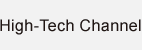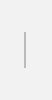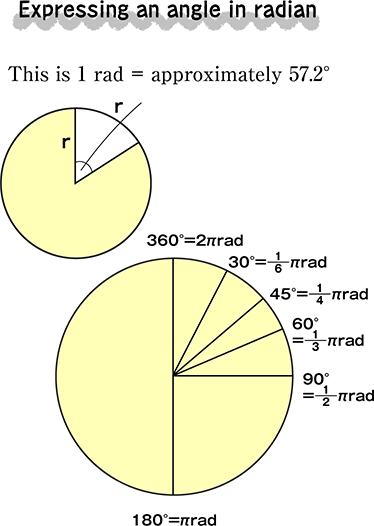### Method of more logically expressing angles than 360-degree

Symbol: rad / Base data: Angle. SI derived units.
Size of central angle for an arc equal to the radius of the circle

Called “circular measure” in Japan

The angle formed at the center of a circle and arc equal in length to the radius of the circle is “1 radian”. For example, 360 degrees is 2 π radian. The　“°” (degree) according to the familiar sexagesimal system is something divided by humans, but this could be called more logical as it is expressed constantly regardless of how you define a circle.